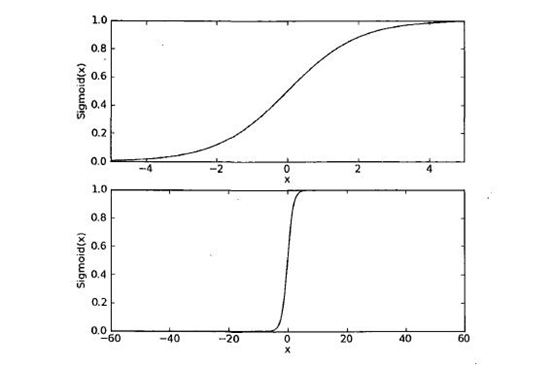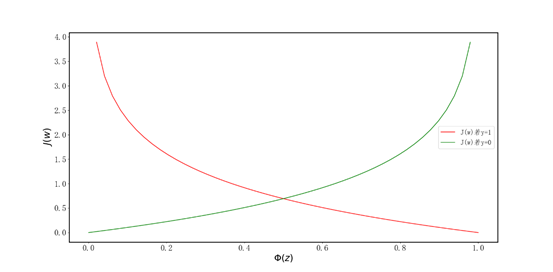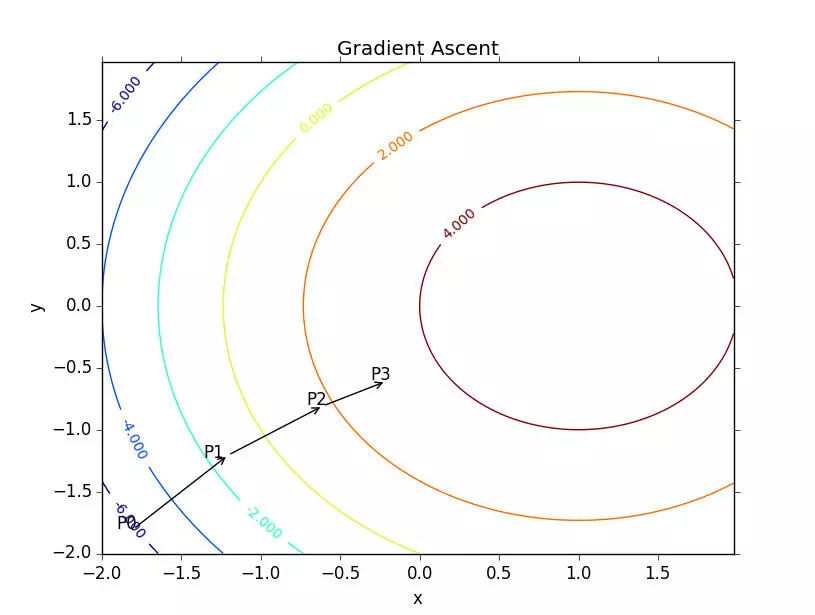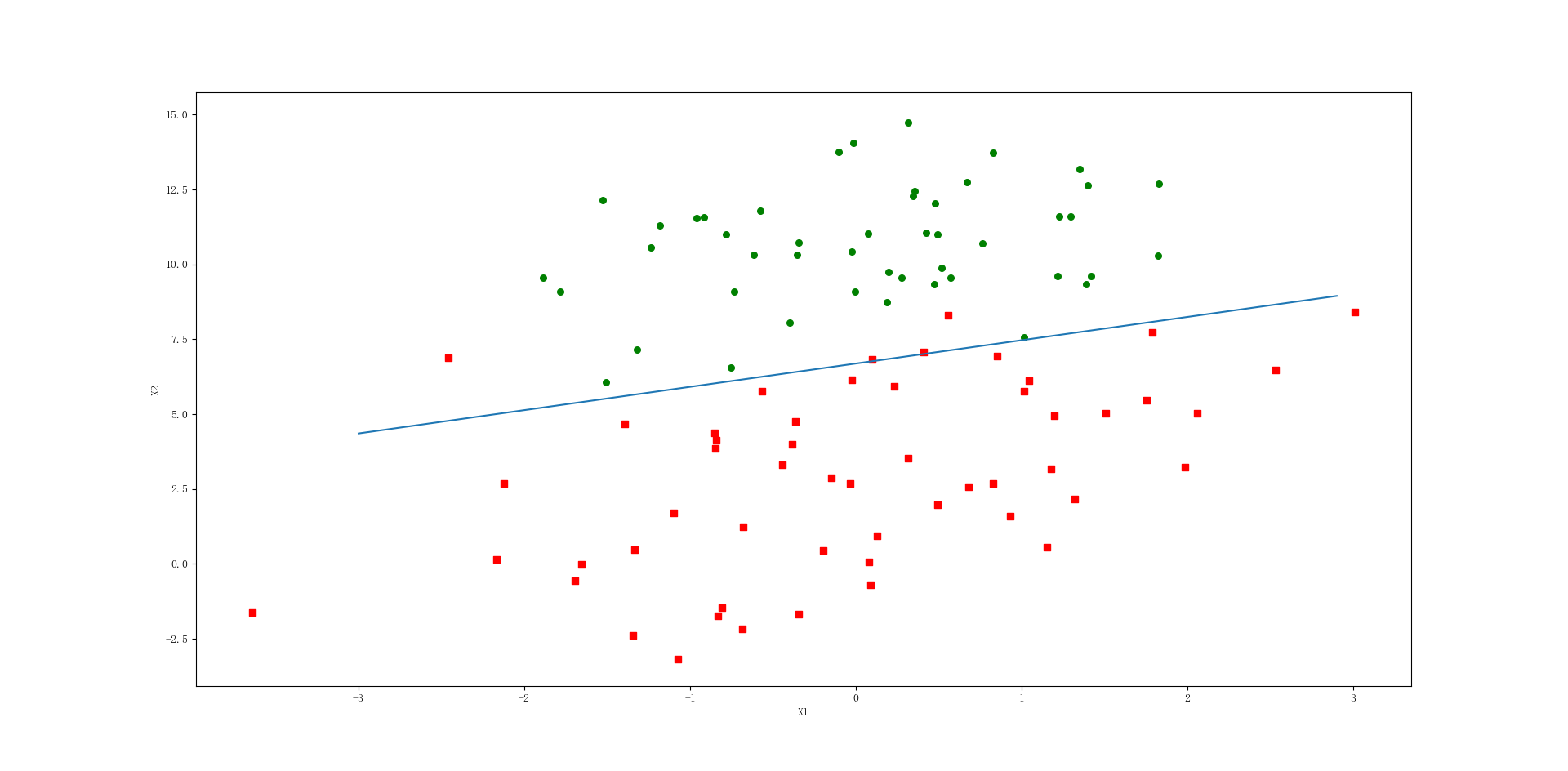# logistic回归详解

逻辑斯谛回归（logistic regression）是统计学习中的经典分类方法，属于对数线性模型，所以也被称为对数几率回归。这里要注意，虽然带有回归的字眼，但是该模型是一种分类算法，逻辑斯谛回归是一种线性分类器，针对的是线性可分问题。利用logistic回归进行分类的主要思想是：根据现有的数据对分类边界线建立回归公式，以此进行分类。这里的“回归”一词源于最佳拟合，表示要找到最佳拟合参数集，因此，logistic训练分类器时的做法就是寻找最佳拟合参数，使用的是最优化方法，接下来我们都会讲到。

#### 一、Logistic回归函数：

我们先说一个概念，事件的几率（odds），是指该事件发生的概率与该事件不发生的概率的比值。如果事件发生的概率是p，那么该事件的几率是p/(1-p)。取该事件发生几率的对数，定义为该事件的对数几率（log odds）或logit函数： log ⁡ i t ( p ) = log ⁡ p 1 − p \log i t(p)=\log \frac{p}{1-p}        看了几个博客，我对其取对数的理解大概是这样：事件发生的概率p的取值范围为[0,1]，对于这样的输入，计算出来的几率只能是非负的（大家可以自己验证），而通过取对数，便可以将输出转换到整个实数范围内，下面是log函数的在二维坐标系中的图像，依照图像就会对标黄的那句话有一个形象的了解了。

那我们将输出转换到整个实数范围内的目的是什么呢？因为这样，我们就可以将对数几率记为输入特征值的线性表达式 ： log ⁡ i t ( p ( y = 1 ∣ x ) ) = w 0 x 0 + w 1 x 1 + … + w n x n = ∑ i = 0 n w i x i = w T x \log i t(p(y=1 | x))=w_{0} x_{0}+w_{1} x_{1}+\ldots+w_{n} x_{n}=\sum_{i=0}^{n} w_{i} x_{i}=w^{T} x        其中，p(y =1|x)是条件概率分布，表示当输入为x时，实例被分为1类的概率，依据此概率我们能得到事件发生的对数几率。但是，我们的初衷是做分类器，简单点说就是通过输入特征来判定该实例属于哪一类别或者属于某一类别的概率。所以我们取logit函数的反函数，令 w T x w^{T}x 的线性组合为输入，p为输出，经如下推导
log ⁡ p 1 − p = w T x 　 　 　 　 　 　 　 　 　 \log \frac{p}{1-p}=w^{T} x　　　　　　　　　 令 w T x = z 　 　 对 上 述 公 式 取 反 　 　 　 令w^{T} x=z 　　对上述公式取反　　　 则 有 ϕ ( z ) = p = 1 1 + e − z 　 　 　 （ １ ） 则有\phi(z)=p=\frac{1}{1+e^{-z}} 　　　（１）那么对于公式1，我们可以这样解释：为了实现logistic回归分类器，我们可以在每个特征上都乘以一个回归系数，然后把所有的结果值相加，将这个总和带入sigmoid函数中。进而得到一个范围在0-1之间的数值。最后设定一个阈值，在大于阈值时判定为1，否则判定为0。以上便是逻辑斯谛回归算法是思想，公式就是分类器的函数形式。

#### 二、最佳回归系数的确定（极大似然估计+最优化方法）

上一节我们已经确定了logisti分类函数，有了分类函数，我们输入特征向量就可以得出实例属于某个类别的概率。但这里有个问题，权重w（回归系数）我们是不确定的。正如我们想的那样，我们需要求得最佳的回归系数，从而使得分类器尽可能的精确。

如何才能获得最佳的回归系数呢？这里就要用到最优化方法。在很多分类器中，都会将预测值与实际值的误差的平方和作为损失函数（代价函数），通过梯度下降算法求得函数的最小值来确定最佳系数。前面我们提到过某件事情发生的概率为p，在逻辑斯蒂回归中所定义的损失函数就是定义一个似然函数做概率的连乘，数值越大越好，也就是某个样本属于其真实标记样本的概率越大越好。如，一个样本的特征x所对应的标记为1,通过逻辑斯蒂回归模型之后，会给出该样本的标记为1和为-1的概率分别是多少，我们当然希望模型给出该样本属于1的概率越大越好。既然是求最大值，那我们用到的最优化算法就是梯度上升，其实也就是与梯度下降相反而已。

1、我们需要先定义一个最大似然函数L，假定数据集中的每个样本都是独立的，其计算公式如下：
L ( w ) = P ( y ∣ x ; w ) = ∏ i = 1 n P ( y ( i ) ∣ x ( i ) ; w ) = ( ϕ ( z ( i ) ) ) y ( i ) ( 1 − ϕ ( z ( i ) ) ) 1 − y ( i ) L(w)=P(y | x ; w)=\prod_{i=1}^{n} P\left(y^{(i)} | x^{(i)} ; w\right)=\left(\phi\left(z^{(i)}\right)\right)^{y^{(i)}}\left(1-\phi\left(z^{(i)}\right)\right)^{1-y^{(i)}}
L(w)就是我们以上说的对于损失函数的最原始的定义，但我们还要进一步处理，那就是取对数，在进行极大似然估计的时候我们都知道要取对数，那为什么我们要取对数呢？

2、取似然函数的对数：
J ( w ) = log ⁡ ( L ( w ) ) = ∑ i = 1 n y ( i ) log ⁡ ( ϕ ( z ( i ) ) ) + ( 1 − y ( i ) ) log ⁡ ( 1 − ϕ ( z ( i ) ) ) J(w)=\log (L(w))=\sum_{i=1}^{n} y^{(i)} \log \left(\phi\left(z^{(i)}\right)\right)+\left(1-y^{(i)}\right) \log \left(1-\phi\left(z^{(i)}\right)\right)

J ( w ) = log ⁡ ( L ( w ) ) = ∑ i = 1 n − y ( i ) log ⁡ ( ϕ ( z ( i ) ) ) − ( 1 − y ( i ) ) log ⁡ ( 1 − ϕ ( z ( i ) ) ) J(w)=\log (L(w))=\sum_{i=1}^{n} -y^{(i)} \log \left(\phi\left(z^{(i)}\right)\right)-\left(1-y^{(i)}\right) \log \left(1-\phi\left(z^{(i)}\right)\right)

J ( ϕ ( z ) , y ; w ) = − y log ⁡ ( ϕ ( z ) ) − ( 1 − y ) log ⁡ ( 1 − ϕ ( z ) ) J(\phi(z), y ; w)=-y \log (\phi(z))-(1-y) \log (1-\phi(z))

J ( Φ ( z ) , y ; w ) = { − log ⁡ ( Φ ( z ) ) 若 y = 1 − log ⁡ ( 1 − Φ ( z ) ) 若 y = 0 J(\Phi(z), y ; w)=\left\{\begin{array}{ll}{-\log (\Phi(z))} & {若\mathrm{y}=1} \\ {-\log (1-\Phi(z))} & {若\mathrm{y}=0}\end{array}\right.∂ ∂ w j Ｊ ( w ) = ( − y 1 ϕ ( z ) + ( 1 − y ) 1 1 − ϕ ( z ) ) ∂ ∂ w j ϕ ( z ) \frac{\partial}{\partial w_{j}} Ｊ(w)=\left(-y \frac{1}{\phi(z)}+(1-y) \frac{1}{1-\phi(z)}\right) \frac{\partial}{\partial w_{j}} \phi(z)

∂ ∂ w j ϕ ( z ) = ∂ ∂ z 1 1 + e − z = 1 ( 1 + e − z ) 2 e − z = 1 1 + e − z ( 1 − 1 1 + e − z ) = ϕ ( z ) ( 1 − ϕ ( z ) \frac{\partial}{\partial w_{j}} \phi(z)=\frac{\partial}{\partial z} \frac{1}{1+e^{-z}}=\frac{1}{\left(1+e^{-z}\right)^{2}} e^{-z}=\frac{1}{1+e^{-z}}\left(1-\frac{1}{1+e^{-z}}\right)=\phi(z)(1-\phi(z)

( － y 1 ϕ ( z ) ＋ ( 1 − y ) 1 1 − ϕ ( z ) ) ∂ ∂ w j ϕ ( z ) = ( － y 1 ϕ ( z ) ＋ ( 1 − y ) 1 1 − ϕ ( z ) ) ϕ ( z ) ( 1 − ϕ ( z ) ) ∂ ∂ w j z = ( － y ( 1 − ϕ ( z ) ) ＋ ( 1 − y ) ϕ ( z ) ) x j = － ( y − ϕ ( z ) ) x j \begin{array}{l}{　\left(－y \frac{1}{\phi(z)}＋(1-y) \frac{1}{1-\phi(z)}\right) \frac{\partial}{\partial w_{j}} \phi(z)} \\ {=\left(－y \frac{1}{\phi(z)}＋(1-y) \frac{1}{1-\phi(z)}\right) \phi(z)(1-\phi(z)) \frac{\partial}{\partial w_{j}} z} \\ {=(－y(1-\phi(z))＋(1-y) \phi(z)) x_{j}} \\ {=－(y-\phi(z)) x_{j}}\end{array}

w j : = w j － η ∑ i = 1 n ( y ( i ) − ϕ ( z ( i ) ) ) x ( i ) w_{j} :=w_{j}－\eta \sum_{i=1}^{n}\left(y^{(i)}-\phi\left(z^{(i)}\right)\right) x^{(i)}

w : = w － Δ w w :=w－\Delta w

Δ w j = − η ∂ J ∂ w j = η ∑ i = 1 n ( y ( i ) − ϕ ( z ( i ) ) x ( i ) ) w : = w + Δ w , Δ w = − η ∇ J ( w ) \begin{aligned} \Delta w_{j} &=-\eta \frac{\partial J}{\partial w_{j}}=\eta \sum_{i=1}^{n}\left(y^{(i)}-\phi\left(z^{(i)}\right) x^{(i)}\right) \\ w & :=w+\Delta w, \Delta w=-\eta \nabla J(w) \end{aligned}

#### 三、梯度上升算法（机器学习实战）

∇ f ( x , y ) = ( ∂ f ( x , y ) ∂ x ∂ f ( x , y ) ∂ y ) \nabla f(x, y)=\left( \begin{array}{c}{\frac{\partial f(x, y)}{\partial x}} \\ {\frac{\partial f(x, y)}{\partial y}}\end{array}\right)上图中的梯度上升算法沿梯度方向移动了一步。可以看到，梯度算子总是指向函数值增长最快的方向。这里所说的是移动方向，而未提到移动量的大小。该量值称为步长，记作 α \alpha 。用向量来表示的话，梯度上升算法的迭代公式如下：
w : = w + α ∇ w f ( w ) w :=w+\alpha \nabla_{w} f(w)
该公式将一直迭代执行，直至达到某个停止条件为止，b比如迭代次数达到某个值或者算法达到某个可以允许的误差范围。

w : = w − α ∇ w f ( w ) w :=w-\alpha \nabla_{w} f(w)

#### 四、一个简单的算法

// An highlighted block
from numpy import *

def loadDataSet():
dataMat = [];
labelMat = []
fr = open('testSet.txt')
for line in fr.readlines():
lineArr = line.strip().split()
dataMat.append([1.0, float(lineArr), float(lineArr)])
labelMat.append(int(lineArr))
return dataMat, labelMat

def sigmoid(inX):
return 1.0 / (1 + exp(-inX))

def gradAscent(dataMatIn, classLabels):
dataMatrix = mat(dataMatIn)  # convert to NumPy matrix
labelMat = mat(classLabels).transpose()  # convert to NumPy matrix
m, n = shape(dataMatrix)
alpha = 0.001
maxCycles = 500
weights = ones((n, 1))
for k in range(maxCycles):  # heavy on matrix operations
h = sigmoid(dataMatrix * weights)  # matrix mult
error = (labelMat - h)  # vector subtraction
weights = weights + alpha * dataMatrix.transpose() * error  # matrix mult
return weights

def plotBestFit(weights):
import matplotlib.pyplot as plt
dataMat, labelMat = loadDataSet()
dataArr = array(dataMat)
n = shape(dataArr)
xcord1 = [];
ycord1 = []
xcord2 = [];
ycord2 = []
for i in range(n):
if int(labelMat[i]) == 1:
xcord1.append(dataArr[i, 1]);
ycord1.append(dataArr[i, 2])
else:
xcord2.append(dataArr[i, 1]);
ycord2.append(dataArr[i, 2])
fig = plt.figure()
ax = fig.add_subplot(111)
ax.scatter(xcord1, ycord1, s=30, c='red', marker='s')
ax.scatter(xcord2, ycord2, s=30, c='green')
x = arange(-3.0, 3.0, 0.1)
y = (-weights - weights * x) / weights  #最佳拟合直线
ax.plot(x, y)
plt.xlabel('X1');
plt.ylabel('X2');
plt.show()

if __name__ =='__main__':
dataArr,labelMat=loadDataSet()
weights=gradAscent(dataArr,labelMat)
plotBestFit(weights.getA())分类效果还是很不错的，当然这只是一个简单的实现，具体的优化算法等大家可以看一些书籍和博客上都有介绍。本人所了解到的也就这么多，有什么说的不对的地方望见谅，欢迎指正。

1、《机器学习实战》［美］Peter Harrington 著
2、《统计学习方法》 李航 著
3、《机器学习》 周志华 著
4、https://blog.csdn.net/sinat_29957455/article/details/78944939
5、https://blog.csdn.net/gwplovekimi/article/details/80288964#commentBo

05-0639811-1022万+
04-145万+
06-0510万+
06-065万+
07-1629万+
12-231622
10-194755
11-3010万+
12-11747
©️2020 CSDN 皮肤主题: 大白 设计师:CSDN官方博客